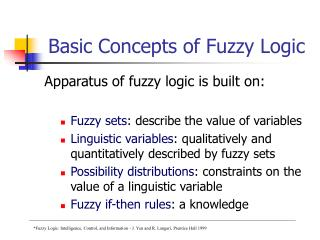Download PresentationBasic Concepts of Fuzzy Logic

# Basic Concepts of Fuzzy Logic - PowerPoint PPT PresentationDownload Presentation## Basic Concepts of Fuzzy Logic

- - - - - - - - - - - - - - - - - - - - - - - - - - - E N D - - - - - - - - - - - - - - - - - - - - - - - - - - -
##### Presentation Transcript

1. Basic Concepts of Fuzzy Logic Apparatus of fuzzy logic is built on: • Fuzzy sets: describe the value of variables • Linguistic variables: qualitatively and quantitatively described by fuzzy sets • Possibility distributions: constraints on the value of a linguistic variable • Fuzzy if-then rules: a knowledge *Fuzzy Logic: Intelligence, Control, and Information - J. Yen and R. Langari, Prentice Hall 1999

2. Linguistic variables A fuzzy set can be used to describe the value of a variable. • Temperature is high. • The load is heavy.

3. Linguistic variables The variable Temperature (x) is characterized both by a symbolic variable (“High”) and a numeric variable expressing its membership in the fuzzy set “High”.

4. Linguistic variables A linguistic variable is “a variable whose values are words or sentences in a natural or artificial language”. Each linguistic variable may be assigned one or more linguistic values, which are in turn connected to a numeric value through the mechanism of membership functions.

5. Linguistic variables An example of a fuzzy linguistic variable and membership functions

6. Possibility Distribution Assigning a fuzzy set to a linguistic variable constrains the value of the variable. Possible vs. Impossible values of the variable are a matter of degree.

7. Possibility Distribution Example: a suspect is a male between 20 and 30 years old. A crisp set defines the age of this suspect as [20,30]. A 19-years old male would not be a suspect, as this age is an impossible value for this set.

8. Possibility Distribution A fuzzy set defines the age of this suspect as (age) that may have a smooth boundary.

9. Possibility Distribution A possibility that the suspect is 19 years old is 0.75

10. Possibility Distribution In general, when we assign a fuzzy set A to a variable X, the assignment results in a possibility distribution of X, which is defined by A’s membership function. X(x)=A(x)

11. If-Then rules “If temperature is hot then AC_setting is high” Provide fuzzy inference. Can be viewed as: - Interpolation scheme - Multi-expert panel - Generalization of logic inference

12. If-Then rules “If temperature is hot then AC_setting is high” Provide fuzzy inference. Can be viewed as: - Multi-expert panel - Interpolation scheme - Generalization of logic inference

13. If-Then rules Multi-expert panel: A kingdom with 3 mathematicians. 1. Can sqrt numbers between 0 and 1000 2. Can sqrt numbers between 1001 and 2000 3. Can sqrt numbers between 2001 and 5000 The task: What is the sqrt of 1156.

14. If-Then rules M1: 31.6 How sure? – 0 M2: 34 How sure? – 1 M3: 44.73 How sure? =0 The answer – 34.

15. If-Then rules Interpolation:

16. If-Then rules Inference: Rule: if a person’s income is more than 100K then the person is rich Fact: Jack’s income is 101K Consequence: Jack is rich

17. If-Then rules Structure of fuzzy rules: IF <antecedent> THEN <consequent> Example: IF a person’s income is high THEN the person is rich

18. If-Then rules An antecedent may combine multiple conditions using logic connectives (AND, OR, NOT): IF a person’s income is high AND the income figure is true THEN the person is rich

19. If-Then rules Consequent • Crisp: IF … THEN y=nonfuzzy_value • Fuzzy: IF …THEN y is A_fuzzy_set • Functional: IF x1 is A1 AND x2 is A2 …AND xn is An THEN